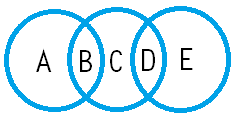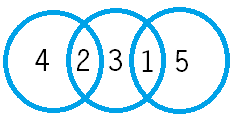## 三個圓圈五個數字快問快答謎題（或整個水平翻轉過來：51324亦可）

#### 解析

zoo2000210:

IF:A=3 E=4 則 沒有解                 (4=2+2，4=1+3 但是因為不可重複所以沒有解)
IF:A=4 E=3 則 沒有解                 (理由同上)
IF:A=3 E=5 則 B=4 C=1 D=2      (5=2+3，5=1+4 但是因為不可重複因此選擇1+4)
IF:A=5 E=3 則 B=2 C=1 D=4      (理由同上)
IF:A=4 E=5 則 B=2 C=3 D=1      (4=2+2，4=1+3 但是因為不可重複因此選擇1+3)
IF:A=5 E=4 則 B=1 C=3 D=2      (理由同上)
yaonepiece:

1~5總合為15，而因為三個圈圈的和應要一樣，所以(A+B)+(B+C+D)+(D+E)應該要被3整除。整理得(A+B+C+D+E)+(B+D)。

3：
(B,D)為(1,2)或(2,1)，而每個圈的總合為(15+3)/3=6。將剩下的數字填入後就是腦補的兩個答案。
6：
(B,D)為(1,5)、(5,1)、(2,4)或(4,2)，而每個圈的總合為(15+6)/3=7。將剩下的數字填入後會得到(5,2,1,4,3)和(3,4,1,2,5)兩解。
9：
(B,D)為(4,5)或(5,4)，而每個圈的總合為(15+9)/3=8。但此種無解。

10,480